Download PDF文章快速检索 高级检索

, 王延奎1,2, 沙永祥2 , 王磊2
1. 空气动力学国家重点实验室, 绵阳 621000;
2. 北京航空航天大学 航空科学与工程学院, 北京 100083

Effects of perturbation geometry on behavior of asymmetric flow over blunt body
QI Zhongyang1,2, WANG Yankui1,2, SHA Yongxiang2 , WANG Lei2
1. State Key Laboratory of Aerodynamics, Mianyang 621000, China ;
2. School of Aeronautic Science and Engineering, Beijing University of Aeronautics and Astronautics, Beijing 100083, China
Received: 2015-12-29; Accepted: 2016-04-01; Published online: 2016-05-23 08:57
Foundation item: National Natural Science Foundation of China (11472028); China Aerospace Science and Technology Corporation Innovation Fund (CASC01); Pre-research Fund 2015
Corresponding author. Tel.:010-82339591, E-mail:wangyankui@buaa.edu.cn
Abstract: The asymmetric vortices can be determined through setting the artificial perturbation on the nose of the blunt body at high angle of attack. To study the influence of perturbation geometry on the asymmetric vortices, numerical simulation was applied and the hemispherical, D-type and square perturbations were set on the position circumferential angle 90° and meridian angle 10° respectively at the angle of attack 50° and ReD=1.54×105. It is found that the vortex structure induced by hemispherical perturbation is shown as right vortex pattern; however the left vortex pattern is shown for the D-type and square perturbations. What is more, the asymmetry of vortex structure for the square perturbation is weaker than that for the other two perturbations. The reason is that the separated flows from different boundaries of the same perturbation influence each other and affect the asymmetric vortex structure. In order to determine the asymmetric vortices accurately by setting artificial perturbation, the geometry of perturbation should be as simple as possible.
Key words: blunt body     asymmetric vortices     artificial perturbation     perturbation geometry     high angle of attack

1 研究方法 1.1 数值方法

1.2 物理模型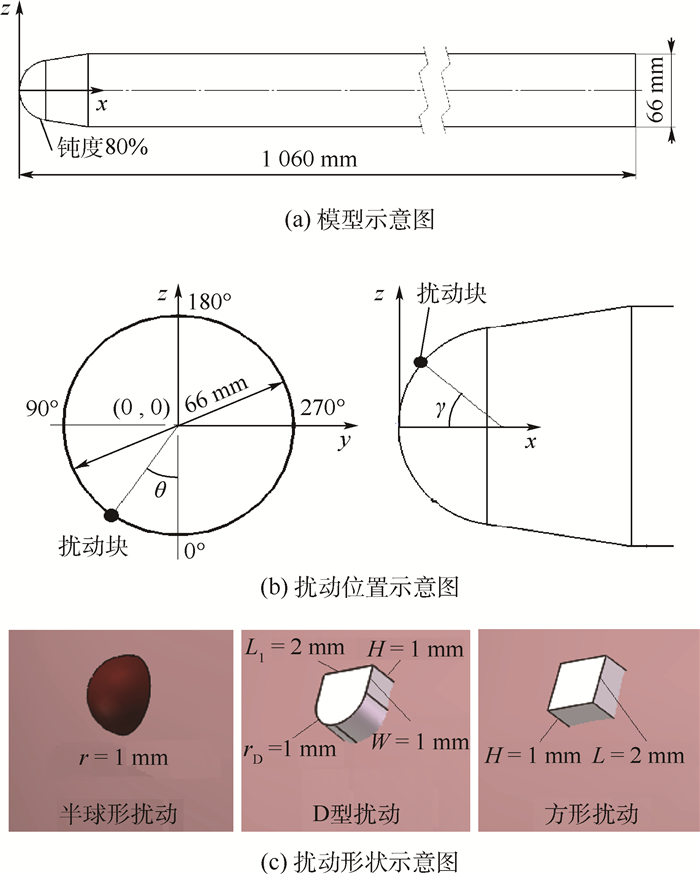图 1 模型与人工扰动 Fig. 1 Model and artificial perturbation

1.3 数值方法验证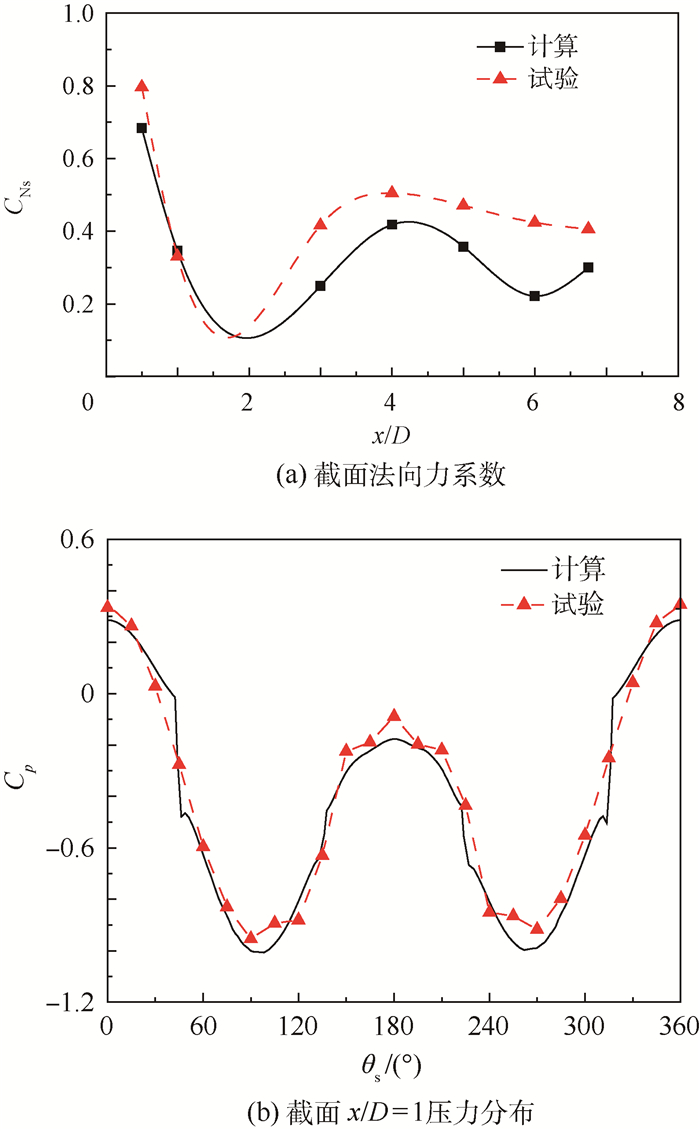图 2 数值方法验证(V∞=35 m/s，α=40°，θ=90°，γ=40°，ReD=1.54×105) Fig. 2 Verification of numerical simulation method (V∞=35 m/s, α=40°, θ=90°, γ=40°, ReD=1.54×105)
2 结果与分析 2.1 扰动形状对表面压力分布的影响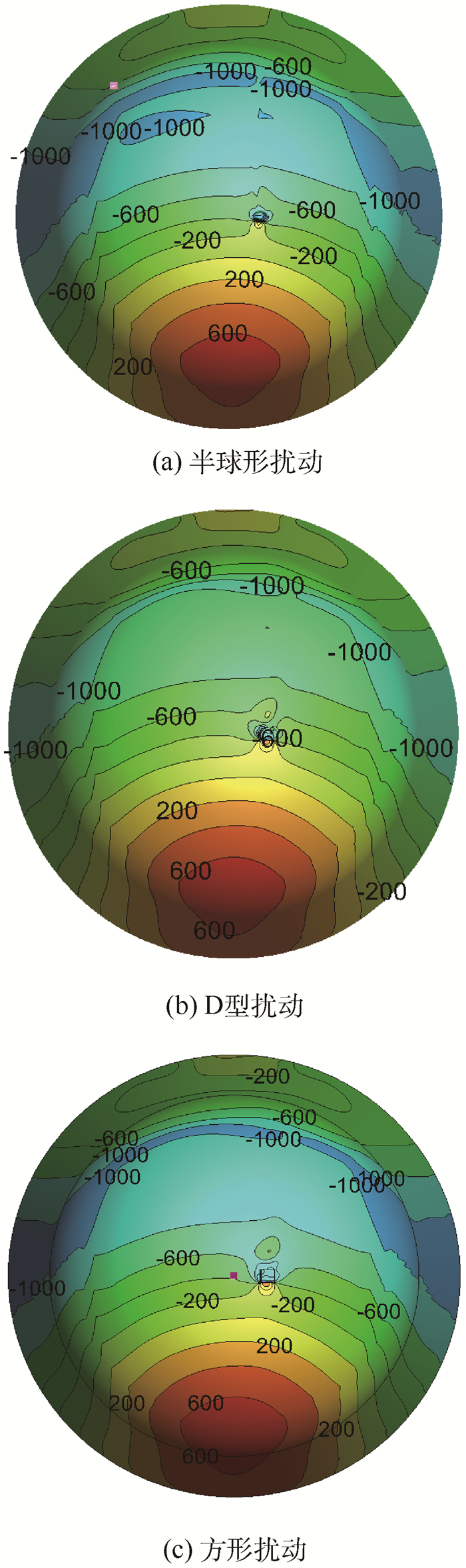图 3 3种扰动块下钝头体头部压力分布(V∞=35 m/s，α=50°，θ=90°，γ=10°，ReD=1.54×105) Fig. 3 Nose pressure distribution of blunt body for three perturbations (V∞=35 m/s, α=50°, θ=90°, γ=10°, ReD=1.54×105)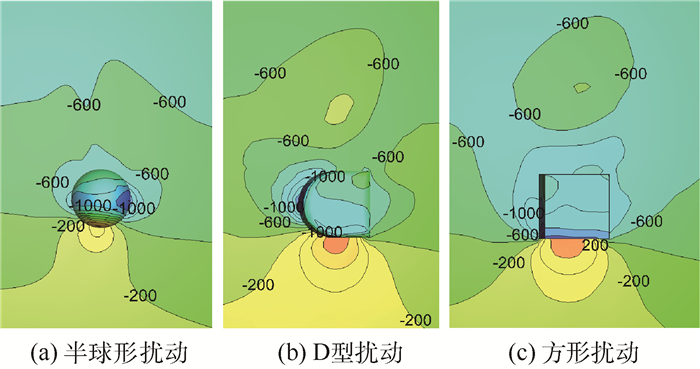图 4 3种扰动块下扰动位置的压力分布(V∞=35 m/s，α=50°，θ=90°，γ=10°，ReD=1.54×105) Fig. 4 Locational pressure distribution for three perturbations (V∞=35 m/s, α=50°, θ=90°, γ=10°, ReD=1.54×105)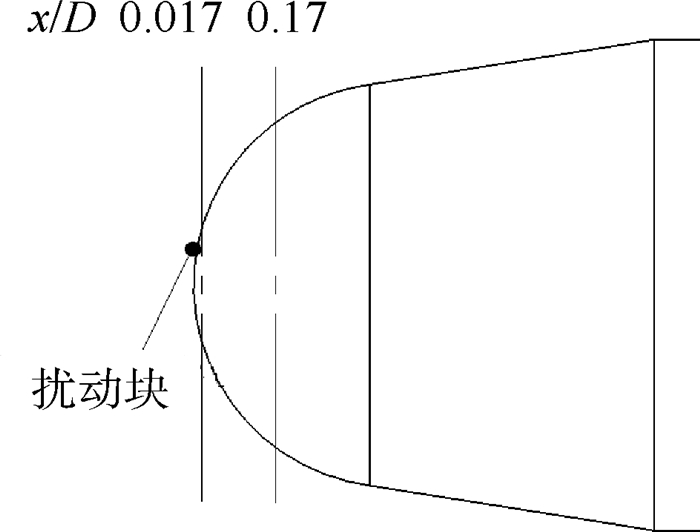图 5 模型头部压力分析截面 Fig. 5 Nose sections of model for pressure analysis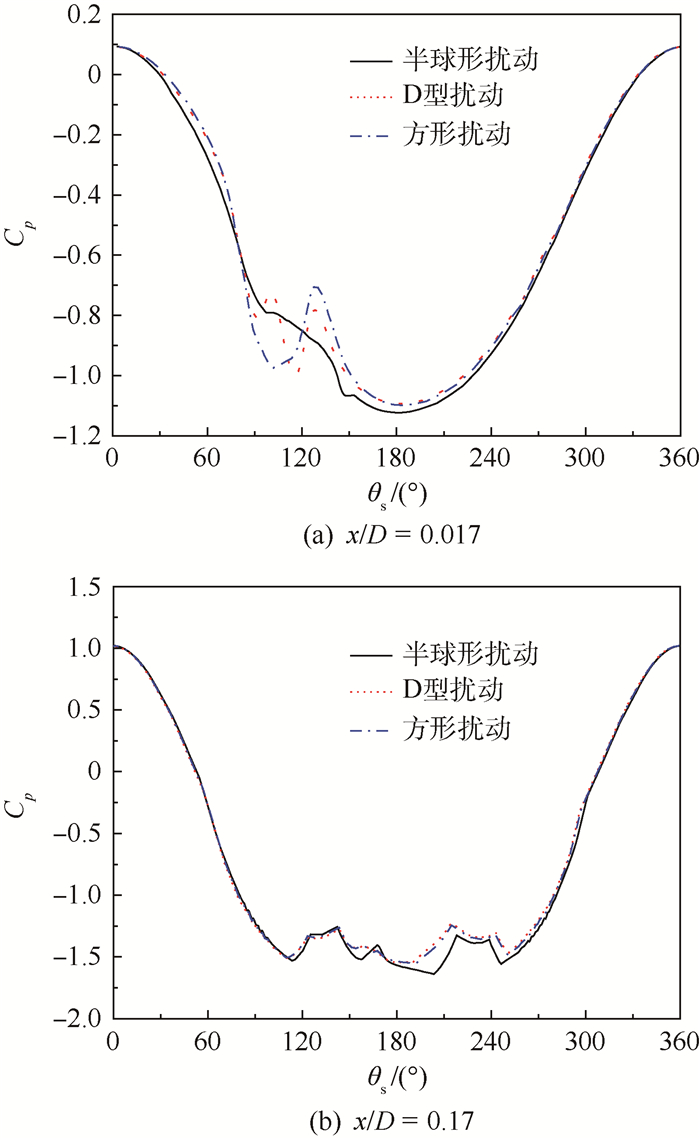图 6 3种扰动块下模型头部截面的压力系数分布(V∞=35 m/s，α=50°，θ=90°，γ=10°，ReD=1.54×105) Fig. 6 Sectional pressure coefficient distribution of model nose for three perturbations (V∞=35 m/s, α=50°, θ=90°, γ=10°, ReD=1.54×105)

2.2 扰动形状对流场结构的影响

2.2.1 扰动形状对钝头体非对称流场的影响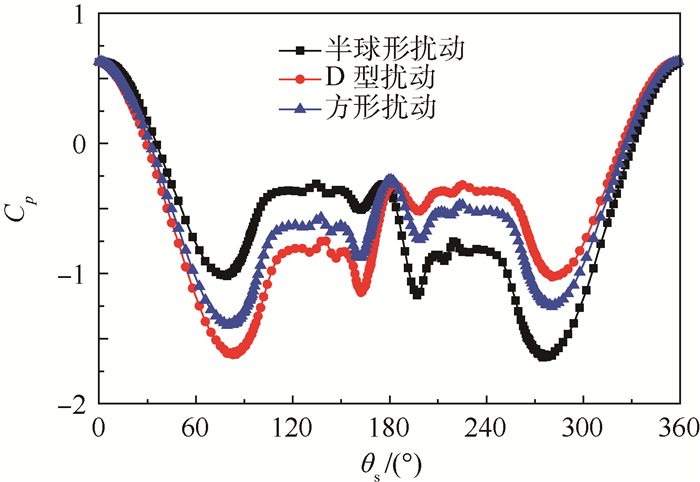图 7 3种扰动块下截面x/D=2.5的压力系数分布(V∞=35 m/s，α=50°，θ=90°，γ=10°，ReD=1.54×105) Fig. 7 Pressure coefficient distribution for three perturbations at section x/D=2.5 (V∞=35 m/s, α=50°, θ=90°, γ=10°, ReD=1.54×105)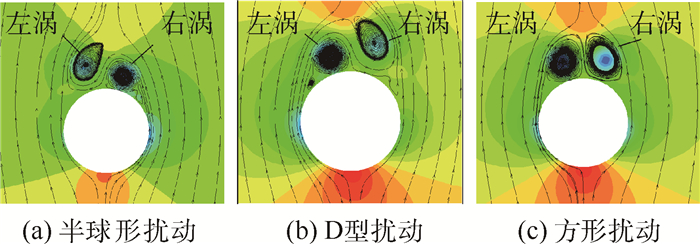图 8 3种扰动块下截面x/D=2.5的压力分布与流线(V∞=35 m/s，α=50°，θ=90°，γ=10°，ReD=1.54×105) Fig. 8 Pressure distribution and streamlines for three perturbations at section x/D=2.5 (V∞=35 m/s, α=50°, θ=90°, γ=10°, ReD=1.54×105)

1)半球形扰动对流场结构的影响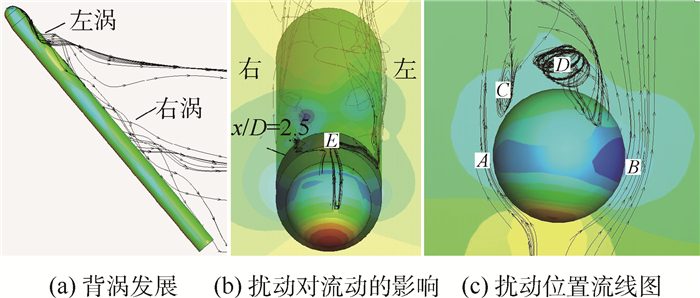图 9 半球形扰动对背涡结构的影响 Fig. 9 Effect of hemispherical perturbation on vortex structure

2) D型扰动对流场结构的影响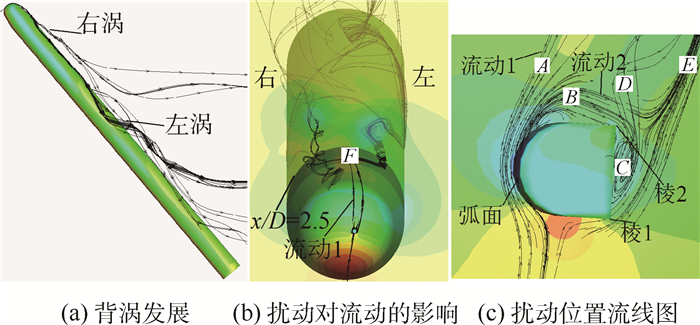图 10 D型扰动对背涡结构的影响 Fig. 10 Effect of D-type perturbation on vortex structure

3)方形扰动对流场结构的影响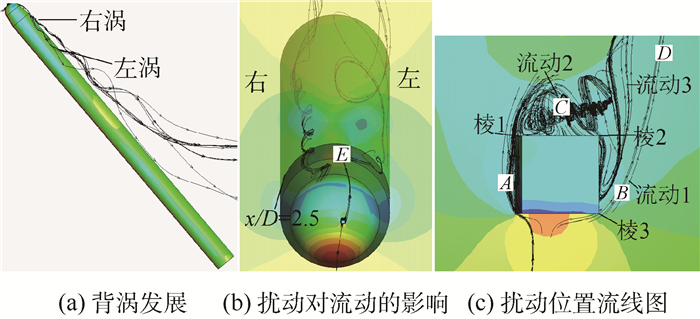图 11 方形扰动对背涡结构的影响 Fig. 11 Effect of square perturbation on vortex structure

2.2.2 扰动形状对钝头体表面流动的影响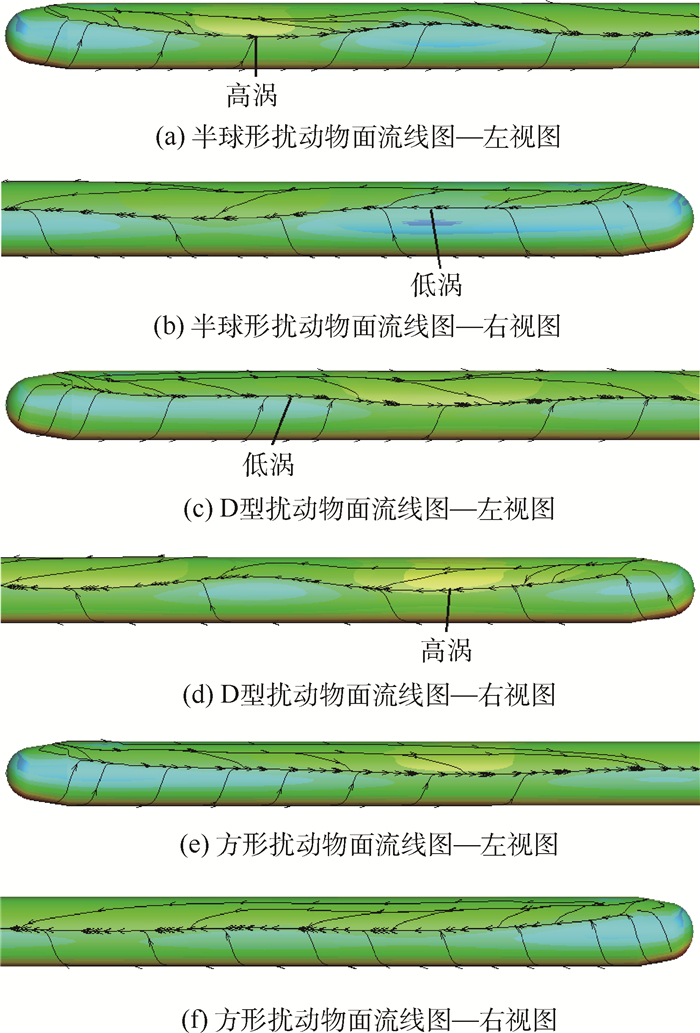图 12 物面分离线 Fig. 12 Separation lines of model surface

3 结论

1) 3种扰动块形状对于钝头体的头部表面压力分布影响主要集中于扰动块周围的背风区域，随着流动向下游发展，其对头部靠近下游的位置影响减小。

2)扰动块对钝头体大攻角的非对称流动有主控作用，且扰动块所引起的微流动的x轴涡旋向决定钝头体的非对称的左、右涡模态；微流动的涡量决定非对称程度的大小。

  王刚, 梁新刚, 邓学蓥. 细长体大迎角绕流的滚转角特性[J]. 实验流体力学, 2004, 18 (4) : 11 –14. WANG G, LIANG X G, DENG X Y. Effects of roll angle on side force distribution over slender bodies of revolution at high angle of attack[J]. Experiments and Measurements in Fluid Mechanics, 2004, 18 (4) : 11 –14. (in Chinese)  ERICSSON L E, BEYERS M E.Fluid mechanics considerations for successful design of forebody flow control:AIAA-2000-2320[R].Reston:AIAA, 2000.  张涵信, 冉政. 细长锥体有攻角绕流对称流态到非对称流态的结构稳定性研究[J]. 空气动力学学报, 1997, 15 (1) : 20 –26. ZHANG H X, RAN Z. On the structural stability of the flows over slenders at angle of attack[J]. Acta Aerodynamica Sinica, 1997, 15 (1) : 20 –26. (in Chinese)  程克明, 范召林, 尹贵鲁. 大攻角流动非对称性成因与对策[J]. 南京航空航天大学学报, 2002, 34 (1) : 17 –21. CHENG K M, FAN Z L, YIN G L. On cause and research strategy of flow asymmetry in high-alpha flows[J]. Journal of Nanjing University of Aeronautics and Astronautics, 2002, 34 (1) : 17 –21. (in Chinese)  LAMONT P J, HUNT B L. Prediction of aerodynamic out-of-plane forces on ogive-nosed circular cylinders[J]. Journal Space-craft and Rockets, 1997, 14 (1) : 38 –44.  WILLIAMS D A.A review of forebody vortex control scenarios:AIAA-1997-1967[R].Reston:AIAA, 1997.  MALOCOLM G N.Forebody vortex control-A progress review:AIAA-1993-3540[R].Reston:AIAA, 1993.  王元靖, 范召林, 侯跃龙, 等. 粗糙带对细长体大迎角流动非对称性的影响[J]. 空气动力学学报, 2005, 23 (3) : 284 –288. WANG Y J, FAN Z L, HOU Y L, et al. Effects of grid strip on flow asymmetry over a slender revolution body at high angles of attack[J]. Acta Aerodynamica Sinica, 2005, 23 (3) : 284 –288. (in Chinese)  MOSKOVITZ C A, HALL R M, DEJARNETTE F R.Effects of nose bluntness, roughness and surface perturbations on the asymmetric flow past slender bodies at large angles of attack:AIAA-1989-2236[R].Reston:AIAA, 1989.  DRIVER D M.Reynolds shear stress measurements in a separated boundary layer:AIAA-1991-1787[R].Reston:AIAA, 1991.  周宇, 钱炜褀, 邓有奇, 等. κ-ω SST两方程湍流模型中参数影响的初步分析[J]. 空气动力学学报, 2010, 28 (2) : 213 –217. ZHOU Y, QIAN W Q, DENG Y Q, et al. Introductory analysis of the influence of menter's κ-ω SST turbulence model's parameters[J]. Acta Aerodynamica Sinica, 2010, 28 (2) : 213 –217. (in Chinese)  MENTER F R.Zonal two equation κ-ω turbulence models for aerodynamic flows:AIAA-1993-2906[R].Reston:AIAA, 1993.  邓学蓥, 刘沛青, 孔繁美. 旋成体非对称背涡特性及其形成机理的研究[J]. 空气动力学学报, 2000, 18 (S1) : 121 –126. DENG X Y, LIU P Q, KONG F M. Investigation on characteristics of asymmetric vortices and mechanism[J]. Acta Aerodynamica Sinica, 2000, 18 (S1) : 121 –126. (in Chinese)

#### 文章信息

QI Zhongyang, WANG Yankui, SHA Yongxiang, WANG Lei

Effects of perturbation geometry on behavior of asymmetric flow over blunt body

Journal of Beijing University of Aeronautics and Astronsutics, 2016, 42(12): 2691-2697
http://dx.doi.org/10.13700/j.bh.1001-5965.2015.0861